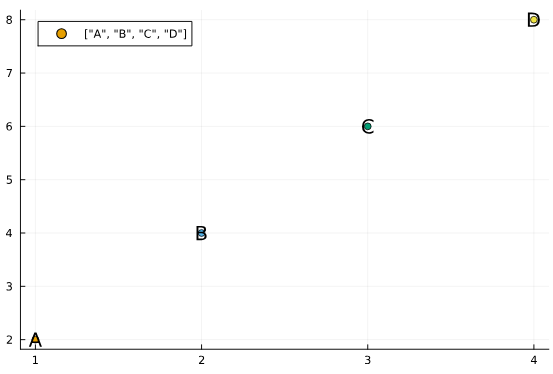# When using seriestype=:scatter, create individual labels for each dot?

I am using Plot recipes to create a plot overload for a special type of input.

The result is a scatter plot with something like:

``````@recipe function f(ms::MyStruct)
seriestype --> :scatter
ms.x_vals, ms.y_vals
end
``````

How do I create individual labels for each of the dots? If I do e.g.

``````label --> "Dot " .* string.(1:length(ms.x_vals))
``````

I get a single label like `["Dot 1", "Dot 2", ...]`. Is there a way around it? I could do it with several plot commands (`plot` followed by `plot!`), but I am not sure how to combine this with plot recipes.

`series_annotations`

1 Like

That put the labels on the dots themselves, but I just want an ordinary label, e.g.:

``````plot(1:4, 2:2:8, seriestype=:scatter; series_annotations=["A", "B", "C", "D"], label=["A", "B", "C", "D"], color=1:4)
``````

givesbut I want something likeTranspose the vector of labels.

1 Like

You need each point to be it’s own series to do that, that’s the internal logic. To create new series in a recipe, use the `@series` macro:

``````@recipe function f(ms::MyStruct)
seriestype --> :scatter
for i in eachindex(ms.x_vals)
@series begin
label := "Dot \$i"
ms.x_vals[i:i], ms.y_vals[i:i]
end
end
end
``````
2 Likes

Thanks a lot, that works